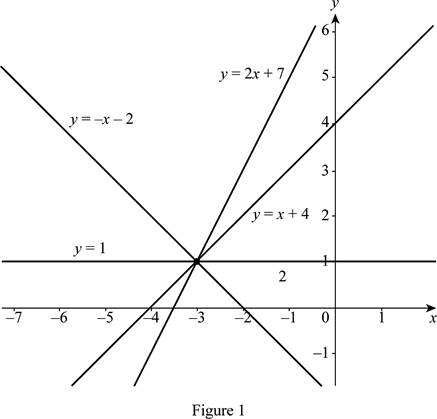# The common members of the family of linear function f ( x ) = 1 + m ( x + 3 ) ; sketch and mark the members on the graph.### Single Variable Calculus: Concepts...

4th Edition
James Stewart
Publisher: Cengage Learning
ISBN: 9781337687805### Single Variable Calculus: Concepts...

4th Edition
James Stewart
Publisher: Cengage Learning
ISBN: 9781337687805

#### Solutions

Chapter 1.2, Problem 6E
To determine

## To find: The common members of the family of linear function f(x)=1+m(x+3) ; sketch and mark the members on the graph.

Expert Solution

The members of the family of a linear function f(x)=1+m(x+3) pass through the common point (−3, 1).

### Explanation of Solution

Consider the linear function y=1+m(x+3) .

Recall the fact that the slope (m) of the function is the coefficient of the x term and y-intercept (c) is the constant term of the function.

Obtain the values of y-intercepts for the various m values.

If m=1 , then c=4 .

If m=1 , then c=2 .

If m=0 , then c=1 .

If m=2 , then c=7 .

Substitute the above values of m and corresponding values of c in the linear function y=mx+c and draw the graph of them as shown below in Figure 1.From Figure 1, it is noticed that all the members of the family of a linear function f(x)=1+m(x+3) passes through the common point (−3, 1).

### Have a homework question?

Subscribe to bartleby learn! Ask subject matter experts 30 homework questions each month. Plus, you’ll have access to millions of step-by-step textbook answers!